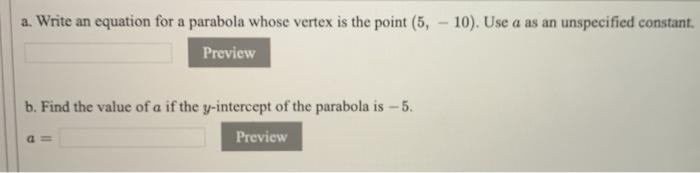# Question a. Write an equation for a parabola whose vertex is the point (5. - 10). Use a as an unspecified constant. Preview b. Find the value of a if the y-intercept of the parabola is -5. Preview